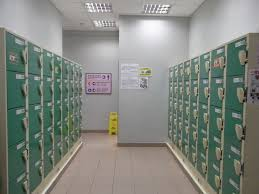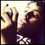# Locker Problem TheoryEvery day, $100$ students enter a school that has $100$ lockers. All the lockers are closed when they arrive.

Student $1$ opens every locker.

Student $2$ closes every second locker.

Student $3$ changes the state of every third locker i.e. he opens it if it is closed and closes it if its open.

Student $4$ changes the state of every fourth locker and so on... so that student $n$ changes the state of every $nth$ locker.

One day several students are absent. Regardless, those present complete the procedure and simply skip the students who are absent. For e.g. if student $3$ is absent, then nobody changes the state of every third locker.

Satyen Nabar discussed the problem when one door was opened and all others were closed.This inspired me and tried to interpret the situation when 2 doors are opened and all the others are closed.

I took two cases

$Case 1:$ Here after 100th operation locker number $1,2$ were opened and all others were closed.I found that $(2*3=6,2*5=10,2*7=14,2*11=22,..........,2*47=94)$and $(8,16,32,64)$,$(9.27,81)$,$(25)$,$(49)$ student nos who were absent.So total $14+4+3+1+1=23$ students were absent.

$Case 2:$In this case after 100th operation locker number $2,4$ were opened and all other were closed.Here I found that $26$ students were present.Student no $2,6,10,14,18,.......,98$ and $4$(which is an exception).So in this case $74$students were absent.

Anyone please confirm these two answers and help me to generalize the problem.Note by Kalpok Guha
6 years, 6 months ago

This discussion board is a place to discuss our Daily Challenges and the math and science related to those challenges. Explanations are more than just a solution — they should explain the steps and thinking strategies that you used to obtain the solution. Comments should further the discussion of math and science.

When posting on Brilliant:

• Use the emojis to react to an explanation, whether you're congratulating a job well done , or just really confused .
• Ask specific questions about the challenge or the steps in somebody's explanation. Well-posed questions can add a lot to the discussion, but posting "I don't understand!" doesn't help anyone.
• Try to contribute something new to the discussion, whether it is an extension, generalization or other idea related to the challenge.

MarkdownAppears as
*italics* or _italics_ italics
**bold** or __bold__ bold
- bulleted- list
• bulleted
• list
1. numbered2. list
1. numbered
2. list
Note: you must add a full line of space before and after lists for them to show up correctly
paragraph 1paragraph 2

paragraph 1

paragraph 2

[example link](https://brilliant.org)example link
> This is a quote
This is a quote
    # I indented these lines
# 4 spaces, and now they show
# up as a code block.

print "hello world"
# I indented these lines
# 4 spaces, and now they show
# up as a code block.

print "hello world"
MathAppears as
Remember to wrap math in $$ ... $$ or $ ... $ to ensure proper formatting.
2 \times 3 $2 \times 3$
2^{34} $2^{34}$
a_{i-1} $a_{i-1}$
\frac{2}{3} $\frac{2}{3}$
\sqrt{2} $\sqrt{2}$
\sum_{i=1}^3 $\sum_{i=1}^3$
\sin \theta $\sin \theta$
\boxed{123} $\boxed{123}$

Sort by:

Sorry to say that your answers are ...inaccurate. in Case 1 it should be 48 and in Case 2 it should be 75 (quite close, though).

I used a PC program to solve, and checked back to confirm. If you wanna the detail result (which student is absent and which isnot), I can provide for you.

I found out that, since the number of combination of lockers' states is $2^n$ and the number of combination of student's states is also $2^n$, no 2 combination of student's states lead to the same result. It's some kind of function. :)

- 6 years, 6 months ago

Thank you very much but please provide the the details.And which function do you used to solve it?

- 6 years, 6 months ago

Brute force. Create 2 arrays: $current$ to store the states of the lockers, and $result$ to store the final state you want in case you have many test cases.

Let $i$ be the counter from 1 to 100. If $current_i\neq result_i$, then $i^{th}$ student goes to school (so he can change his locker's state); also the state of every lockers $j$, which are divisible by $i$, should be altered (close to open and vice versa). If $current_i=result_i$, $i^{th}$ student is absent, since all students after him cannot change his locker's state.

The "states" of students can be stored in another array for recheck.

Here is result for Case 1

- 6 years, 6 months ago

Thank you.Have you got any generalization of this problem?

- 6 years, 6 months ago

Not yet. I can only solve with specific cases.

- 6 years, 6 months ago

The last statement is quite simple to prove mathematically. Do you see how to do that?

Staff - 6 years, 6 months ago

I need a lemma stated that there is an algorithm, so with each combination of the lockers there are at least one combination of the students that satisfies. With that lemma the statement will be easily proved using contradiction.

Fortunately, I described the algorithm in response to Mr. Guha. ;)

- 6 years, 6 months ago

It's great that you are looking at further extensions of this problem.

Note that locker 1 will always remain open, so case 2 cannot be true.

I believe that the answer will depend on which 2 lockers stay open, and you need to look at the factors/multiples of the other (non-1) locker.

Staff - 6 years, 6 months ago

I think if the No.1 student is absent,then locker 1 will not remain open.But I think you are right that I should look on the factors of lockers.

- 6 years, 6 months ago

oh yes indeed. Not sure why, but it didn't cross my mind that he could be absent.

Staff - 6 years, 6 months ago

This is okay but can you help me generalize it?I think I have got line to think but I need the help from you people.

- 6 years, 6 months ago